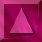Notations

The size of the images is Nx columns by Ny lines.

X is a vector, made from the pixels of an image in a lexicographic order.
Xs is the value of the site (or pixel) s.
Xij is the value of the pixel at column and line j.
Xn is le nth vector of the chain (Xn) .
Let us write P(X) instead of P(x=X).

X0 is the original (non corrupted) image.
Y is the observed data (corrupted image).
H is the convolution operator (NxNy matrix).

Bx, By and W are vectors of the same dimension as X.

h is the generator of H,  it is the Point Spread Function (PSF).

Dx and Dy are the derivative operators applied on the images,
with respect to columns and lines :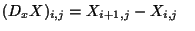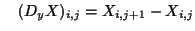dx and dy are the generators of Dx et Dy.

h4 is the generator of H4, H4 is a matrix (2Nx)2(2Ny)2 made by extension of H (padding with zeros).

d4x and d4y are the generators of D4x et D4y, derivative operators applied on symmetrized images whose dimension is 2Nx2Ny.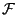represents the Fourier transform.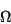is the state space, set of images with dimension NxNy and real pixels, ie.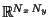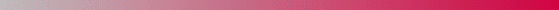André Jalobeanu - 24 Aug 1998